Lennard-Jones model

The Lennard-Jones potential was developed by Sir John Edward Lennard-Jones.

Lennard-Jones potential

The Lennard-Jones potential is given by: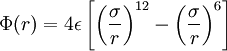$\Phi(r) = 4 \epsilon \left[ \left(\frac{\sigma}{r} \right)^{12}- \left( \frac{\sigma}{r}\right)^6 \right]$

where:

•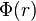$\Phi(r)$ is the intermolecular pair potential between two particles at a distance r;
•$\sigma$ : diameter (length);
•$\epsilon$ : well depth (energy)

Reduced units:

• Density,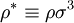$\rho^* \equiv \rho \sigma^3$, where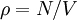$\rho = N/V$ (number of particles$N$ divided by the volume$V$.)
• Temperature;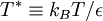$T^* \equiv k_B T/\epsilon$, where$T$ is the absolute temperature and$k_B$ is the Boltzmann constant

Argon

The Lennard-Jones parameters for argon are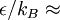$\epsilon/k_B \approx$ 119.8 K and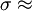$\sigma \approx$ 0.3405 nm. (Ref. ?)

This figure was produced using gnuplot with the command:

plot (4*120*((0.34/x)**12-(0.34/x)**6))

Features

Special points:

•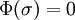$\Phi(\sigma) = 0$
• Minimum value of$\Phi(r)$ at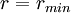$r = r_{min}$;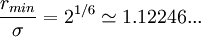$\frac{r_{min}}{\sigma} = 2^{1/6} \simeq 1.12246 ...$

Critical point

The location of the critical point is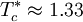$T_c^* \approx 1.33$

at a reduced density of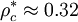$\rho_c^* \approx 0.32$.

Caillol (Ref. 3) reports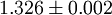$1.326 \pm 0.002$ and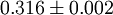$0.316 \pm 0.002$.

Triple point

The location of the triple point as found by Mastny and de Pablo (Ref. 2) is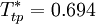$T_{tp}^* = 0.694$

Approximations in simulation: truncation and shifting

The Lennard-Jones model is often used with a cutoff radius of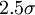$2.5 \sigma$. See Mastny and de Pablo (Ref. 2) fa an analysis of the effect of this cutoff on the melting line.

Related potential models

It is relatively common the use of potential functions given by: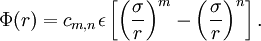$\Phi (r) = c_{m,n} \epsilon \left[ \left( \frac{ \sigma }{r } \right)^m - \left( \frac{\sigma}{r} \right)^n \right].$

with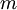$m$ and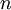$n$ being positive integer numbers and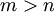$m > n$, and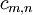$c_{m,n}$ is chosen to get the minimum value of$\Phi(r)$ being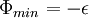$\Phi_{min} = - \epsilon$

These forms are usually referred to as m-n Lennard-Jones Potential.

The 9-3 Lennard-Jones interaction potential is often use to model the interaction between the atoms/molecules of a fluid and a continuous solid wall. In (9-3 Lennard-Jones potential) a justification of this use is presented.